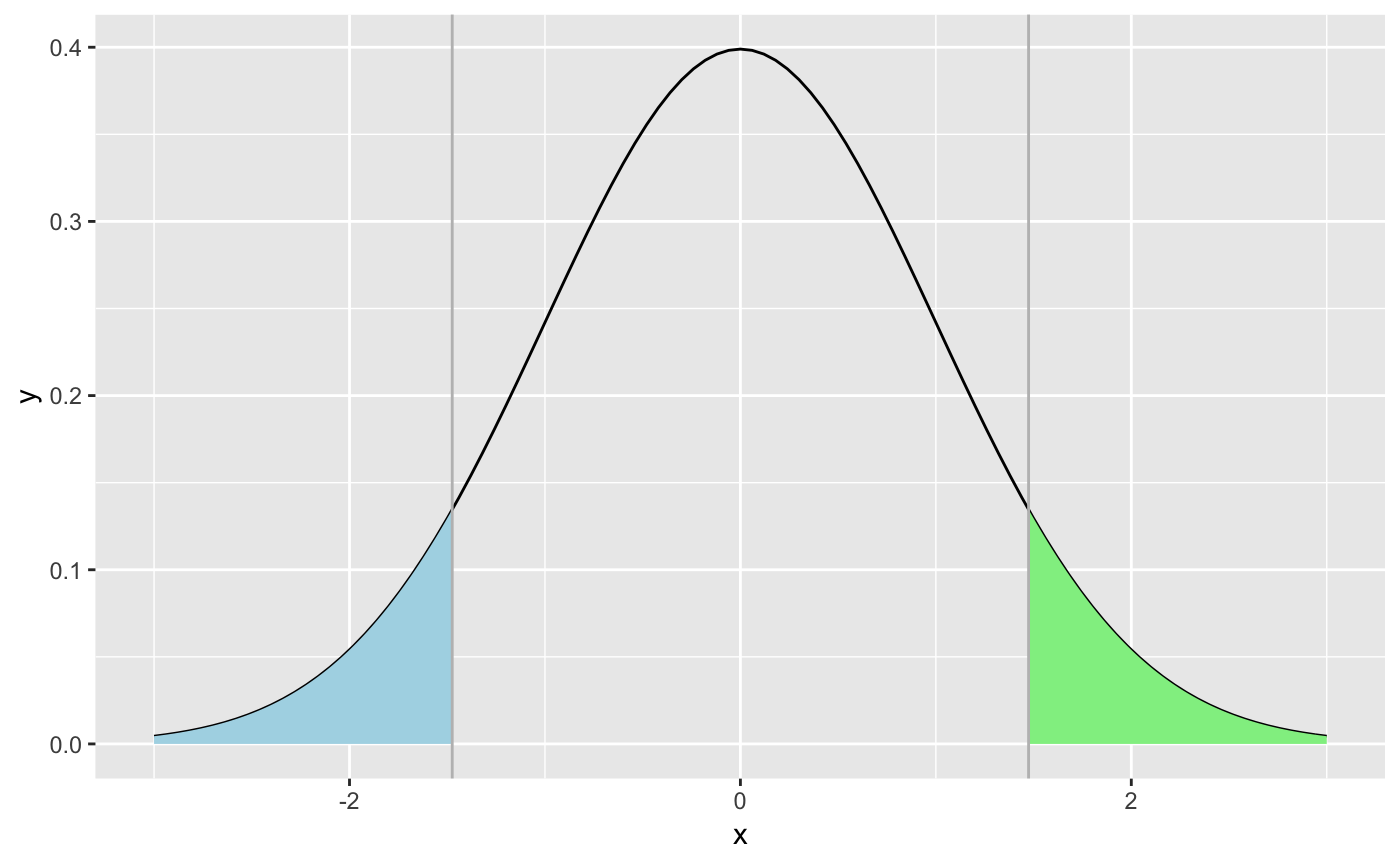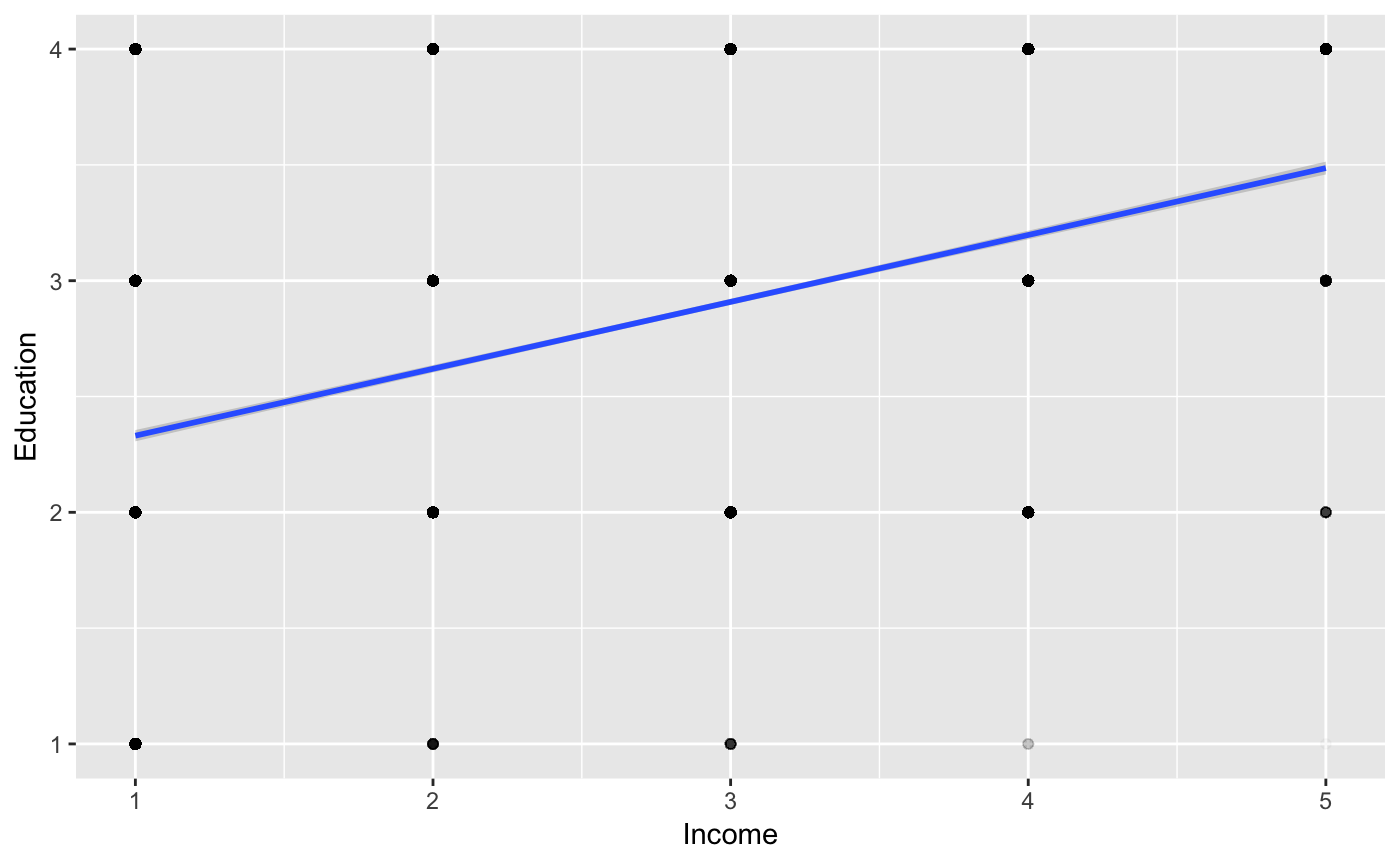This is the companion notebook to the course on Hypothesis testing with application to regression coefficients. We start by testing means, using the ANES database.

library(tidyverse) # Start with the package
load("anes.RData") # Then, change your working directory & load the data

## Testing the mean

### One sample

The simplest and most comprehensible test. We look at the proportion of women among respondents of the surveys.

gender <- anes$Gender %>% as.numeric %>% -1 # Gender is recoded: 0 for male, 1 for female N <- length(gender) # Sample size mean(gender) # Sample mean  0.5360138 m <- 0.53 # Our benchmark, the sample mean => more women! s <- sqrt(0.51*(1-0.51)) # Assuming knowledge of 'true' sd t <- sqrt(N)*(sum(gender)/N-m)/s # Applying the formula ggplot(data.frame(x = c(-3, 3)), aes(x)) + stat_function(fun = dnorm) + stat_function(fun = dnorm, xlim = c(-3,-t), geom = "area", fill = "lightblue") + stat_function(fun = dnorm, xlim = c(t, 3), geom = "area", fill = "lightgreen") + geom_vline(xintercept=-t, color = "grey") + geom_vline(xintercept=t, color = "grey")The shaded zones represent the odds of observing data that would be more extreme (i.e., further from zero), if we assume the null to be true. In this case, under the null, $$\hat{m}=m$$. To get the probability (p-value) associated to t (assuming ‘known’ standard deviation): 2*pnorm(-abs(t)) # For the two-sided test: sample mean != m  0.1403577 pnorm(-abs(t)) # For the one-sided test: sample mean < m  0.07017885 t.test(gender, mu = m) # Using the built-in t-test (Student)  One Sample t-test data: gender t = 1.478, df = 15021, p-value = 0.1394 alternative hypothesis: true mean is not equal to 0.53 95 percent confidence interval: 0.5280380 0.5439896 sample estimates: mean of x 0.5360138  The p-value implies that the mean is not strongly different from 0.53. Nonetheless, the test for pure parity $$m=0.5$$ would show that parity is not reached in this sample. ### Two samples First, a look at the data: we build a pivot table based on years. synth <- anes %>% group_by(Date) %>% summarize(prop = mean(Gender == "Female"), size = n()) # Computes proportions and sample sizes synth Proportions and sample sizes vary across time. Is it likely that the proportions of women respondents in 1996 and 2012 are different? test_stat <- function(m1,m2,n1,n2){ # Creates a function that performs the simple two-mean test (m1-m2)/sqrt(m1*(1-m1)/n1+m2*(1-m2)/n2) } t <- test_stat(synth$prop, synth$prop, synth$size, synth$size) # We apply it on the third and seventh proportions t # Show t  2.914288 2*pnorm(-abs(t)) # p-value  0.003565009 Even at a low significance level, the null hypothesis must be rejected: the two proportions are not equal. Let’s compare the last value (2016) with all others. m <- synth$prop
n <- synth$size test_stat(m,synth$prop,n,synth$size)  1.0532517 1.6739717 -1.2257876 0.5388272 1.3140163 -0.2309392 3.5436459 0.0000000 If we set the threshold to $$|t|> 2$$ (5% significance level), only the 7th survey stands out. This means that the demographic properties of the survey are different, at least at the gender level and comparisons and predictions should account for that. Finally, we look at tests that do not rely on proporions, but general series. Let’s compare the age of women versus the age of men in our sample. We resort to the built-in t.test() function. See below for documentation: https://stat.ethz.ch/R-manual/R-devel/library/stats/html/t.test.html age_men <- filter(anes, Gender == "Male")$Age       # Ages of all men
age_women <- filter(anes, Gender == "Female")$Age # Ages of all women mean(age_men) # Average age of men  47.51808 mean(age_women) # Average age of women  47.99342 t.test(age_men, age_women) # Test: are they equal?  Welch Two Sample t-test data: age_men and age_women t = -1.6889, df = 14812, p-value = 0.09126 alternative hypothesis: true difference in means is not equal to 0 95 percent confidence interval: -1.02701378 0.07633316 sample estimates: mean of x mean of y 47.51808 47.99342  The p-value is rather small, but not significantly enough. We cannot reject the null hypothesis: average ages are not statistically different.. ## Regression analysis We now turn to regression analysis. Our aim is to check if, in our ANES dataset, there is a link between education and income. Since these variables are (ordered) categorical, we must first translate them into number. Luckily, the as.numeric() function does just that. We store these results in a parallel database, called anes2. anes2 <- anes %>% select(-Date, -Race, -Religion, -Nb_children) # New data variable anes2$Income <- anes2$Income %>% as.numeric() # Numerical income category, could use: mutate_if() anes2$Education <- anes2$Education %>% as.numeric() # Numerical education category head(anes2) # A preview of the data anes2 %>% ggplot(aes(x = Income, y = Education)) + geom_point(alpha = 0.01) + geom_smooth(method='lm') # A visual hintlm(Income ~ Education, data = anes2) %>% summary() # The outcome of the regression  Call: lm(formula = Income ~ Education, data = anes2) Residuals: Min 1Q Median 3Q Max -2.3974 -0.9006 0.0994 0.6026 3.0930 Coefficients: Estimate Std. Error t value Pr(>|t|) (Intercept) 1.410192 0.029624 47.60 <2e-16 *** Education 0.496802 0.009903 50.17 <2e-16 *** --- Signif. codes: 0 ‘***’ 0.001 ‘**’ 0.01 ‘*’ 0.05 ‘.’ 0.1 ‘ ’ 1 Residual standard error: 1.065 on 15020 degrees of freedom Multiple R-squared: 0.1435, Adjusted R-squared: 0.1434 F-statistic: 2517 on 1 and 15020 DF, p-value: < 2.2e-16 Because of the categorical nature of data, the relationship is not obvious to discern. Using alpha intensity, we see that the bottom right zone is empty, meaning that there are few (if any) rich respondents with the lowest level of education (grade school or less). In the regression output, the coefficient associated to education is positive and statistically signification (negligible p-value). Hence, the relationship between the two variables seems robust. Note that this is a ‘qualitative’ assessment nonetheless: it does not imply causality, which is plausible in both directions. Finally, note that the R-squared lies around 0.15, which means that the overall quality of the regression is not exceptional. Now, given the richness of the data, we can investigate the joint effect of other variables on income. Why not take into account gender, age and party affiliation? Gender and party affiliation can easily be coded in numerical variables. On the contrary, race and religion cannot be used because they cannot straightforwardly be translated into numbers (they are unordered). One-hot encoding could circumvent this problem, but we leave it as exercise (see the dummies package for one-hot encoding.) anes2$Gender <- as.numeric(anes2$Gender) - 1 # 0 for mean, 1 for women anes2$Party_simple <- as.numeric(anes2\$Party_simple) # 1 for Dems, 2 for Indeps, 3 for GOP
lm(Income ~ Age + Gender + Education + Party_simple, data = anes2) %>% summary()

Call:
lm(formula = Income ~ Age + Gender + Education + Party_simple,
data = anes2)

Residuals:
Min      1Q  Median      3Q     Max
-2.6787 -0.6813  0.1219  0.7083  3.0809

Coefficients:
Estimate Std. Error t value Pr(>|t|)
(Intercept)   1.2693241  0.0439718  28.867   <2e-16 ***
Age           0.0006914  0.0004989   1.386    0.166
Gender       -0.2147323  0.0172904 -12.419   <2e-16 ***
Education     0.4774415  0.0098619  48.413   <2e-16 ***
Party_simple  0.1480913  0.0109607  13.511   <2e-16 ***
---
Signif. codes:  0 ‘***’ 0.001 ‘**’ 0.01 ‘*’ 0.05 ‘.’ 0.1 ‘ ’ 1

Residual standard error: 1.052 on 15017 degrees of freedom
Multiple R-squared:  0.1639,    Adjusted R-squared:  0.1637
F-statistic: 736.1 on 4 and 15017 DF,  p-value: < 2.2e-16

Age is positively linked to income, but not in a forceful fashion. Gender has a negative impact: given our coding, this means that men earn more than women, on average. The corresponding p-value is very small, which highlights that the effect is significant. Education still plays an important role. The t-stat associated to it (almost 48) is still incredibly high, underlying its strong impact on income (its importance was not affected by the introduction of the other variables). Finally, party affiliation is also correlated to income. The more the respondent is to the political right, the higher its average income.

Below, we rapidly check these claims with short pivot tables.

anes %>% group_by(Gender) %>%
summarise(avg_income = mean(as.numeric(Income)))            # Male/Female average income
anes %>% group_by(Education) %>%
summarise(avg_income = mean(as.numeric(Income)))            # Income by education level
anes %>% group_by(Party_simple) %>%
summarise(avg_income = mean(as.numeric(Income)))            # Income by party affiliation

Clearly, the most marked impact is obtained for education.

As a last investgation, we look at what happens with age.

anes2 %>% ggplot(aes(x = Age, y = Income)) + geom_point(alpha = 0.02) + stat_smooth()